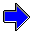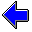Java Security Settings:
This web page employs Java, which requires specific security settings for correct operation.
If the applets on this page do not run correctly, consult the Virtual Chemistry Experiments FAQ
or the Physlet Physics web site for establishing the correct security settings.

# Chemical Kinetics

## Reaction Rates

### Part 1. Concentration Changes

Chemical kinetics is the study of the speed with which a chemical reaction occurs and the factors that affect this speed. This information is especially useful for determining how a reaction occurs.

What is meant by the speed of a reaction? The speed of a reaction is the rate at which the concentrations of reactants and products change.

Consider the following hypothetical example. The letters A, B, and C represent chemical species (in this context, the letters do not represent elements). Suppose the following imaginary reaction occurs:

A + 2 B   →   3 C

The simulation below illustrates how this reaction can be studied. The apparatus at the left is called a stopped-flow apparatus. Each syringe contains a solution filled with a different reactant (A or B). When the two solutions are forced out of the syringes, they are quickly mixed in a mixing block and the reaction starts. The reacting solution passes through the tube at the bottom. An analytical technique such as spectrophotometry is used to measure the concentrations of the species in the reaction mixture (which is in the tube at the bottom) and how those concentrations change with time.

In this example, the syringe at the left contains a solution of species A, which has a green color. The syringe at the right contains a solution of species B, which has a light blue color. The product C has a red color.

The graph at the right shows how the concentration of each species changes as time progresses. Run the simulation and observe the stopped-flow experiment and the shape of the concentration-time plots.

Notice that the color of the reaction mixture changes as the reaction progresses. The reactants are green and light blue, which when mixed produce a green color. As the reactants are consumed, the product, which is red, is produced.

This behavior is reflected in the concentration-time plots. The concentration of A, shown by the green line, decreases as time progresses, because the reaction consumes A. The same behavior is observed for B (light blue line). Conversely, there is initially no product C (red line) present. The reaction produces C, however, so the concentration of C increases as time goes by. (In this simulation, A and B were initially present in stoichiometric amounts.)

### Part 2. Reaction Rate

The rate of change in the concentrations of the reactants and products can be used to characterize the rate of a chemical reaction. The rate of change in the concentration corresponds with the slope of the concentration-time plot.

The simulation below is the same as that presented above except that the slope of the concentration-time curves are also plotted on the graph. Select the species (A, B, or C) whose slope is shown and use the contols to step through the points on the graph.

In examining the simulation, answer the following questions:

• How does the slope change with time for a given species?
• Is the slope the same for all species?
• What is the sign of the slope for the reactants?
• What is the sign of the slope for the product?

Species
A
B
C

 time sec concentration mole -1 slope mole L-1 sec-1

Rate of ReactionChemical Kinetics Home PageVirtual Chemistry Home Page

ReactionRates.html version 2.1
© 2000-2014 David N. Blauch